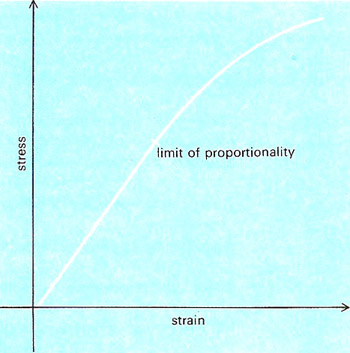A# Hooke's lawPart of the stress/strain curve for a typical metal. Hooke's law applies only below the limit of proportionality.

Hooke's law states that the deformation or strain (change in dimensions) occurring in an elastic body under stress is proportional to the applied stress (force per unit area). The law, which holds only approximately, is named after Robert Hooke who discovered it in 1676.

Hooke's law can be expressed as the formula

stress / strain = a constant.

The value of the constant depends on the material and the type of stress. For tensile and compressive forces it is called Young's modulus, E; for shearing forces, the shear modulus, S; and, for forces affecting the volume of the object, the bulk modulus, K.

Hooke's law applies only below the limit of proportionality (see diagram). Above the elastic limit (not the same as the limit of proportionality) deformation becomes plastic instead of elastic and a permanent set remains when the deforming stress is relaxed.